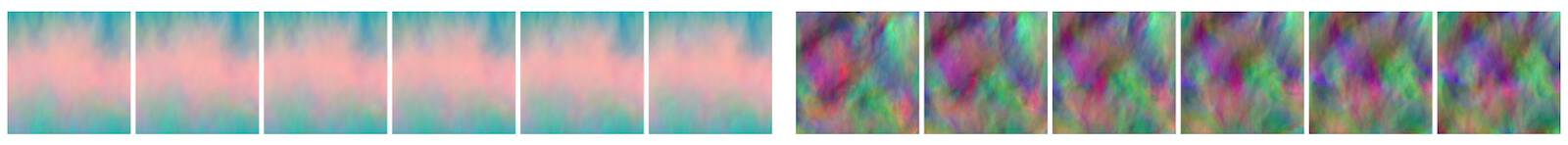# Discussion of Physical Losses#

The good news so far is - we have a DL method that can include physical laws in the form of soft constraints by minimizing residuals. However, as the very simple previous example illustrates, this is just a conceptual starting point.

On the positive side, we can leverage DL frameworks with backpropagation to compute the derivatives of the model. At the same time, this puts us at the mercy of the learned representation regarding the reliability of these derivatives. Also, each derivative requires backpropagation through the full network. This can be very expensive, especially for higher-order derivatives.

And while the setup is relatively simple, it is generally difficult to control. The NN has flexibility to refine the solution by itself, but at the same time, tricks are necessary when it doesn’t focus on the right regions of the solution.

## Is it “Machine Learning”?#

One question that might also come to mind at this point is: can we really call it machine learning? Of course, such denomination questions are superficial - if an algorithm is useful, it doesn’t matter what name it has. However, here the question helps to highlight some important properties that are typically associated with algorithms from fields like machine learning or optimization.

One main reason not to call the optimization of the previous notebook machine learning (ML), is that the positions where we test and constrain the solution are the final positions we are interested in. As such, there is no real distinction between training, validation and test sets. Computing the solution for a known and given set of samples is much more akin to classical optimization, where inverse problems like the previous Burgers example stem from.

For machine learning, we typically work under the assumption that the final performance of our model will be evaluated on a different, potentially unknown set of inputs. The test data should usually capture such out of distribution (OOD) behavior, so that we can make estimates about how well our model will generalize to “real-world” cases that we will encounter when we deploy it in an application.

In contrast, for the PINN training as described here, we reconstruct a single solution in a known and given space-time region. As such, any samples from this domain follow the same distribution and hence don’t really represent test or OOD samples. As the NN directly encodes the solution, there is also little hope that it will yield different solutions, or perform well outside of the training range. If we’re interested in a different solution, we have to start training the NN from scratch.## Summary#

Thus, the physical soft constraints allow us to encode solutions to PDEs with the tools of NNs. An inherent drawback of this variant 2 is that it yields single solutions, and that it does not combine with traditional numerical techniques well. E.g., the learned representation is not suitable to be refined with a classical iterative solver such as the conjugate gradient method.

This means many powerful techniques that were developed in the past decades cannot be used in this context. Bringing these numerical methods back into the picture will be one of the central goals of the next sections.

✅ Pro:

• Uses physical model.

• Derivatives can be conveniently computed via backpropagation.

❌ Con:

• Quite slow …

• Physical constraints are enforced only as soft constraints.

• Largely incompatible with classical numerical methods.

• Accuracy of derivatives relies on learned representation.

To address these issues, we’ll next look at how we can leverage existing numerical methods to improve the DL process by making use of differentiable solvers.